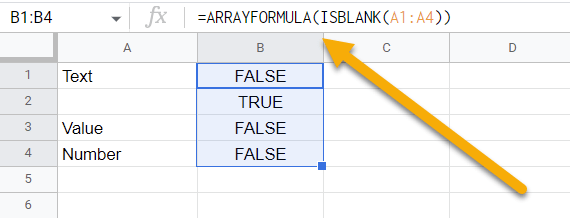# How to☝️ Use the ISBLANK Function in Google Sheets

To use the ISBLANK function in Google Sheets, select a blank cell, go to the Formula bar, enter the formula =ISBLANK(A1), and hit the Enter key.

Continue reading to delve deeper into the topic. Here you will learn the most important details related to the ISBLANK function. Let’s dive into it together!

## How to Quickly Use the ISBLANK Function in Google Sheets

Below you will find a step-by-step guide on how to detect whether a cell contains data or not by using the ISBLANK function.

1. Click on the blank cell where you want the result.
2. Go to the Formula bar and enter the formula =ISBLANK(A1), where A1 is the cell that the formula will check for a value.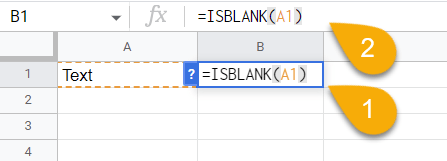1. Lastly, press the Enter key on your keyboard.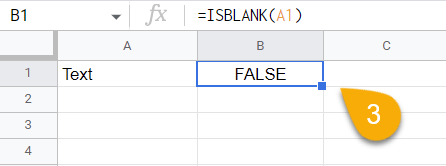Easy as ABC! The formula will confirm whether or not the cell is blank.

## Drilling Down into the ISBLANK Function

The following section contains detailed information regarding the ISBLANK function. Let’s take a look and see what we can learn!

### Description

The ISBLANK function is a built-in function in Google Sheets that is used to check if a cell is empty or not. If the cell is empty, ISBLANK returns TRUE. Otherwise, it returns FALSE.

### Purpose

If you want to quickly determine if a cell is empty or not, particularly when you have a long list of data, you can use this function. By doing this, you will be able to move quickly and easily back and forth between your data and identify the most important elements in your spreadsheet.

=ISBLANK(value)

### Arguments

• value can be a cell reference or another value, such as a range.

### Return Value

In the case of an empty cell, ISBLANK returns TRUE. In any other case, it returns FALSE.

### Usage Notes

Here we present you with some notes to keep in mind while working with the ISBLANK function:

1. If the cell contains no characters other than a space (so it looks blank but technically is not), the value will still be converted to FALSE.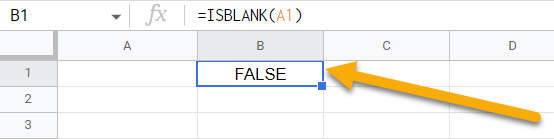1. If a cell contains an error warning, you will get the value FALSE when you execute the operation.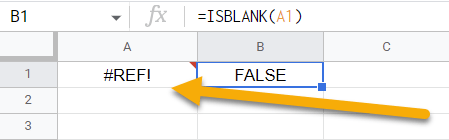1. Not only does ISBLANK allow you to determine the content of a single cell, but it can also be used to determine the contents of a range or set of cells as well.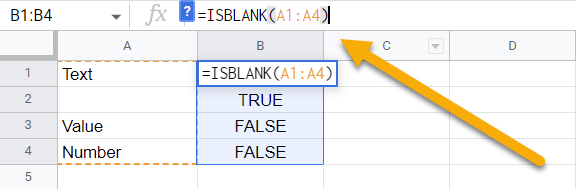### Examples

See some examples below of how you can use this function to your advantage.

1. Use the following formula to determine if a single cell is blank or not:

=ISBLANK(A1) // Output: FALSE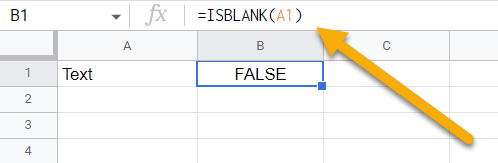1. If you would like to test the contents of a set or range of cells to determine if any of them are blank, use this formula:

=ISBLANK(A1:A4) // Output: FALSE, TRUE, FALSE, FALSE1. You can also apply the ARRAY formula with the ISBLANK function:

=ARRAYFORMULA(ISBLANK(A1:A7)) // Output: FALSE, TRUE, FALSE, FALSE